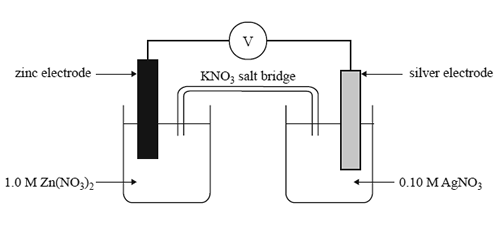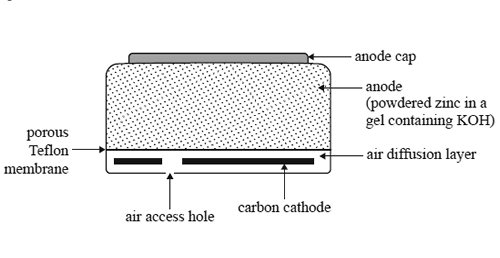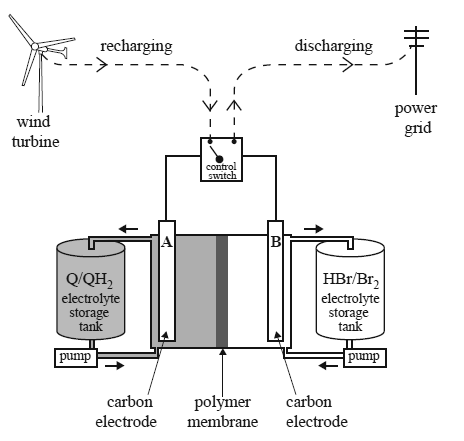General (2014 VCE)1) Which one of the following statements about the cell above is true as the cell discharges? A. The silver electrode is the anode. B. The concentration of Zn2+ ions will increase. C. The maximum voltage delivered by this cell will be 1.56 V. D. Electrons in the external circuit will fl ow from the silver electrode to the zinc electrode. Solution2) What should be observed at the zinc electrode as the cell discharges? A. No change will be observed at this electrode. B. The electrode will become thinner and pitted. C. Crystals will form over the surface of the electrode. D. Bubbles of gas will form over the surface of the electrode. Solution3) The following diagram shows a cross-section of a small zinc-air button cell, a button cell that is used in hearing aids.The zinc acts as the anode. It is in the form of a powder dispersed in a gel (a jelly-like substance) that also contains potassium hydroxide. The cathode consists of a carbon disc. Oxygen enters the cell via a porous Tefl on membrane. This membrane also prevents any chemicals from leaking out. The following reaction takes place as the cell discharges. 2Zn(s) + O2(g) + 2H2O(l) => 2Zn(OH)2(s) a. Write a balanced half-equation for the reaction occurring at the anode. Solutionb. Suggest one role of potassium hydroxide in this cell. Solution Examiner's commentc. A zinc-air button cell is run for 10 hours at a steady current of 2.36 mA. What mass of zinc metal reacts to form zinc hydroxide?   Solution4)Consider the farms. The batteries are recharged using electricity generated by the wind turbines or solar cells. A scientific report, published in January 2014, described a redox flow battery that used a family of chemicals commonly occurring in plants such as rhubarb. These are organic and are known as quinones and hydroquinones. A diagram showing how such a redox flow battery might operate is provided below. In the diagram, Q represents the quinone and QH2 represents the corresponding hydroquinone. The researchers made a model of the redox flow battery using aqueous solutions of the redox pairs, Q/QH2 and Br2/Br–. Refer to the diagram above. During discharge, QH2 is converted to Q and Br2 is converted to HBr. a. Write balanced half-equations for the reactions occurring at the positive and negative electrodes as the cell is discharged. Assume the electrolytes are acidic. Positive electrode :___________________________________ Negative electrode:___________________________________ Solutionb. Write an overall equation for the reaction that occurs when the cell is recharged. Solutionc. The researchers reported that their tests indicated that only hydrogen ions were able to move through the polymer membrane separating the cells. i. Use an arrow (→ or ←) to indicate the direction of movement of hydrogen ions as the cell is recharged. Solution ii. Why is it important that the other reactants in the half-cells are not able to pass through the polymer? Solutiond.The researchers also reported that the voltage applied to the cell during recharging was kept below 1.5 V to avoid the electrolysis of water. Write an equation for the overall reaction that occurs when water is electrolysed. Solution